• 描绘一个数值变量相对于第二个数值变量的变化趋势，在折线图中，每个唯一 x 值或 x 值的分组区间仅绘制一个点（就像直方图一样）。如果一个 x 分组区间中有多个观测值，那么该点在折线图中绘制的 y 值将为该数据点在...
1、作用
描绘一个数值变量相对于第二个数值变量的变化趋势，在折线图中，每个唯一 x 值或 x 值的分组区间仅绘制一个点（就像直方图一样）。如果一个 x 分组区间中有多个观测值，那么该点在折线图中绘制的 y 值将为该数据点在分组中的概括统计值（例如均值或中位数）。绘制的点用线条连接起来，强调 x 值的顺序或相连特性。
2、如果 x 变量表示时间，则数据的折线图通常称之为时间序列图表
3、
# set bin edges, compute centers
xbin_edges = np.arange(0.5, df['num_var1'].max()+0.25, 0.25)
xbin_centers = (xbin_edges + 0.25/2)[:-1]

# 按照xbin_edges分段列的值，返回每段区间内有几个值
data_xbins = pd.cut(df['num_var1'], xbin_edges, right = False, include_lowest = True)
#均值
y_means = df['num_var2'].groupby(data_xbins).mean()
#标准误，不包含缺失值
y_sems = df['num_var2'].groupby(data_xbins).sem()

# plot the summarized data
plt.errorbar(x = xbin_centers, y = y_means, yerr = y_sems)

cut函数
https://www.jianshu.com/p/da693c438316


展开全文数据分析
• #导入包 ...date=xlrd.open_workbook('F:\\study\\python\\AREA.xlsx') #筛选数据 table=date.sheets() x=(1,2,3,4,5,6) y=table.row_values(0) z=table.row_values(1) a=table.row_values(2) b=
#导入包
import xlrd
import os
import matplotlib.pyplot as plt
%matplotlib inline
#打开excel
date=xlrd.open_workbook('F:\\study\\python\\AREA.xlsx')
#筛选数据
table=date.sheets()
x=(1,2,3,4,5,6)
y=table.row_values(0)
z=table.row_values(1)
a=table.row_values(2)
b=table.row_values(3)
#定义画布
plt.figure(dpi=300)
#绘图
plt.plot(x,y,color='b')
plt.plot(x,z,color='g')
plt.plot(x,a,color='r')
plt.plot(x,b,color='y')
plt.xticks([1,2,3,4,5,6],[r'Mid-Holocene',r'Current',r'RCP26',r'RCP40',r'RCP60',r'RCP85'])
o1=plt.plot(x,y,color='b',label='Gansu')
o2=plt.plot(x,z,color='g',label='Qinghai')
o3=plt.plot(x,a,color='r',label='Sichuan')
o4=plt.plot(x,b,color='y',label='Tibet')
plt.legend(bbox_to_anchor=(0.98,1.12),ncol=4)
plt.savefig("熊欢.png")
plt.show()
os.getcwd()#查看存储路径展开全文• import matplotlib.pyplot as plt x = [1,2,3,4,5,6,7,8,9,10,11,12] y = [2,4,3,5,1,6,8,4,6,5,4,9] plt.plot(x,y) plt.show()
import matplotlib.pyplot as plt
x = [1,2,3,4,5,6,7,8,9,10,11,12]
y = [2,4,3,5,1,6,8,4,6,5,4,9]
plt.plot(x,y)
plt.show()




展开全文• 原标题：最适合小白学的花色玩Python折线图|画个天气预报这是菜鸟学Python的第102篇原创文章前面写一篇关于数据可视化的入门开篇，简单的介绍了一下Matplotlib的使用(),今天我们进一步来探索一下Matplotlib到底能画...
原标题：最适合小白学的花色玩Python折线图|画个天气预报这是菜鸟学Python的第102篇原创文章前面写一篇关于数据可视化的入门开篇，简单的介绍了一下Matplotlib的使用(),今天我们进一步来探索一下Matplotlib到底能画哪些图，怎么画呢，其实常见的图形大概6-7种，数据可视化其实除了工具之外，还要思考如何展现，就像画画一下.形固然重要，神更重要.后面我们会详细一一介绍，今天我们先从折线图学起，最后画一个天气预报图要点:简单折线图带刻度和标注的折线图最全的花色折线图带阴影面积的折线图天气预报实战例子1.简单折线图折线图应该算是使用最最频繁的一种图形类型，是用matplotlib.pyplot.plot()函数画的，上一篇其实我们多少提到了一些，下面我们来看看怎么画1). 先来一个最简单的:先创建一个[1,10]的序列作为x轴坐标再创建一个[1,10]的序列的平方为y轴坐标然后plt.figure()算是创建画布最好把x,y轴的序列填入plt.plot()函数中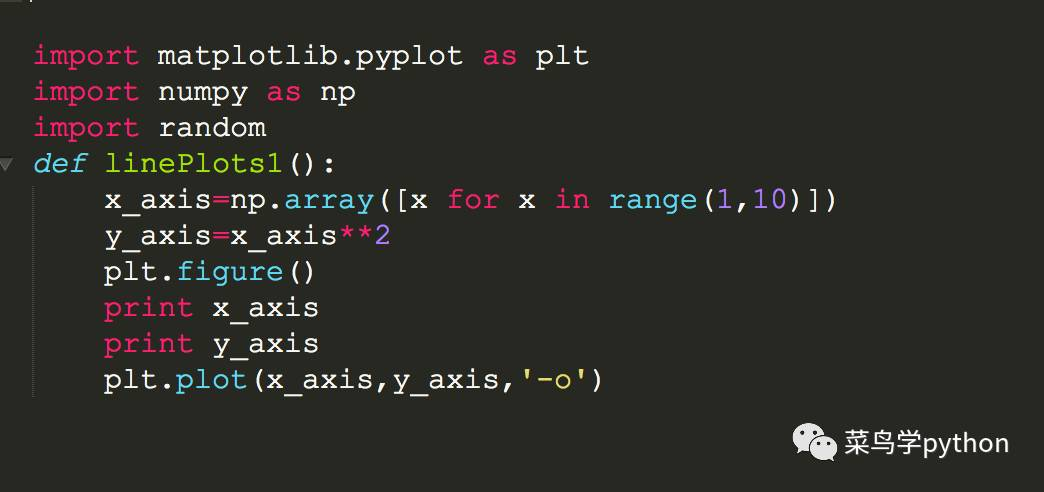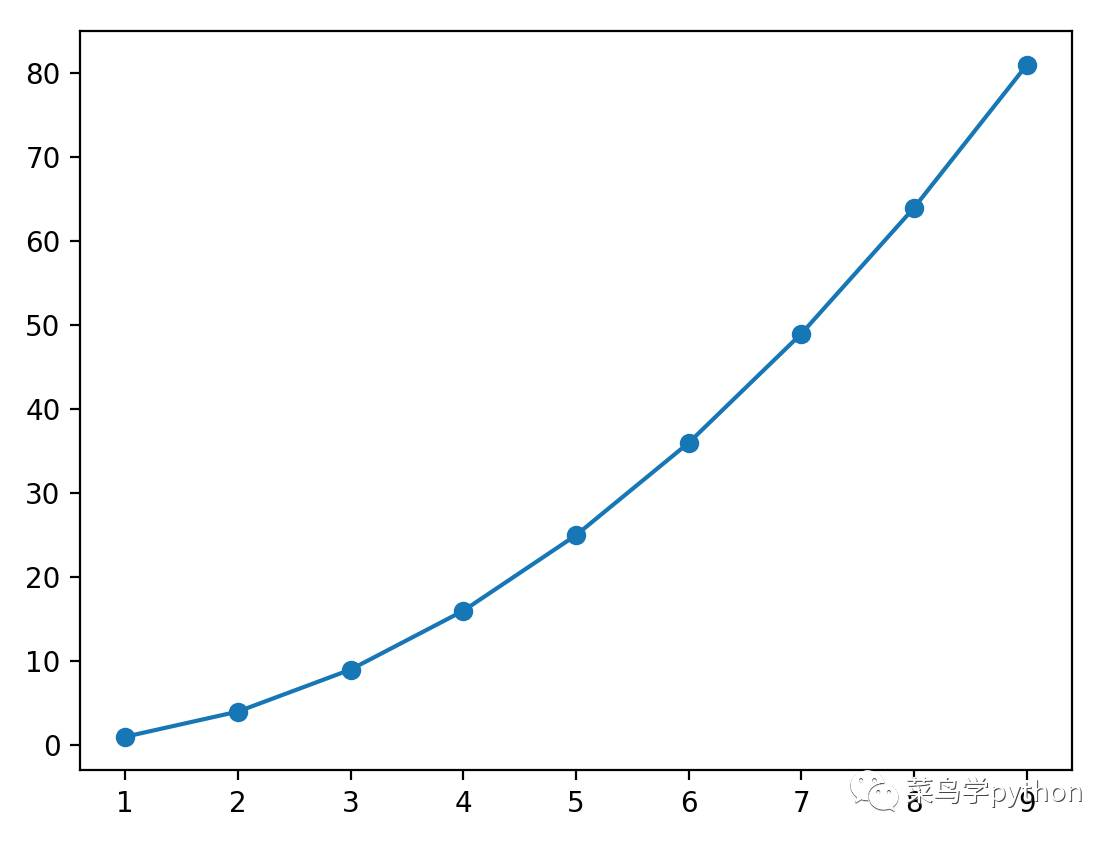是不是很简单啊，上面的图有几个问题：y轴的坐标太粗了，我们希望刻度更精细一点图形曲线上的点能不能标数字好我们慢慢来进化的2.刻度和标注的折线图加y轴坐标和图形数字plt模块里面有一个yticks函数可以自定义y轴的颗粒度然后我plt.gca()获取ax对象循环找到曲线上的坐标点，然后ax.text写入注释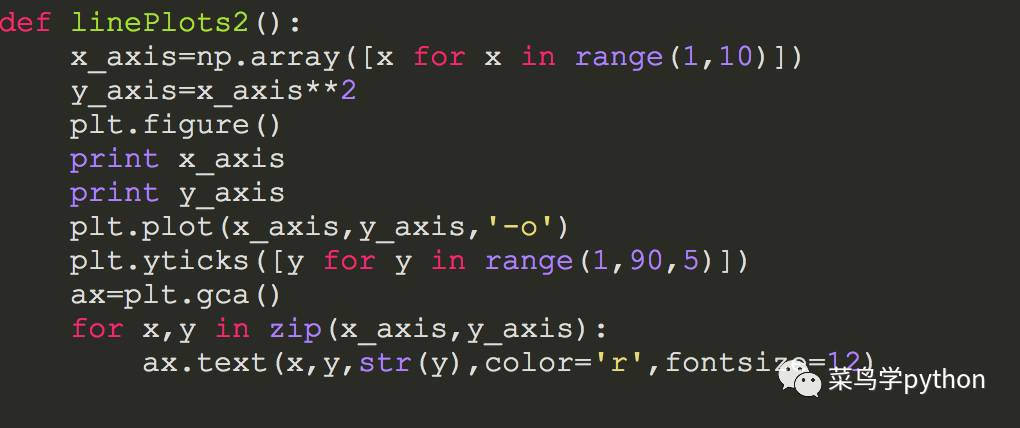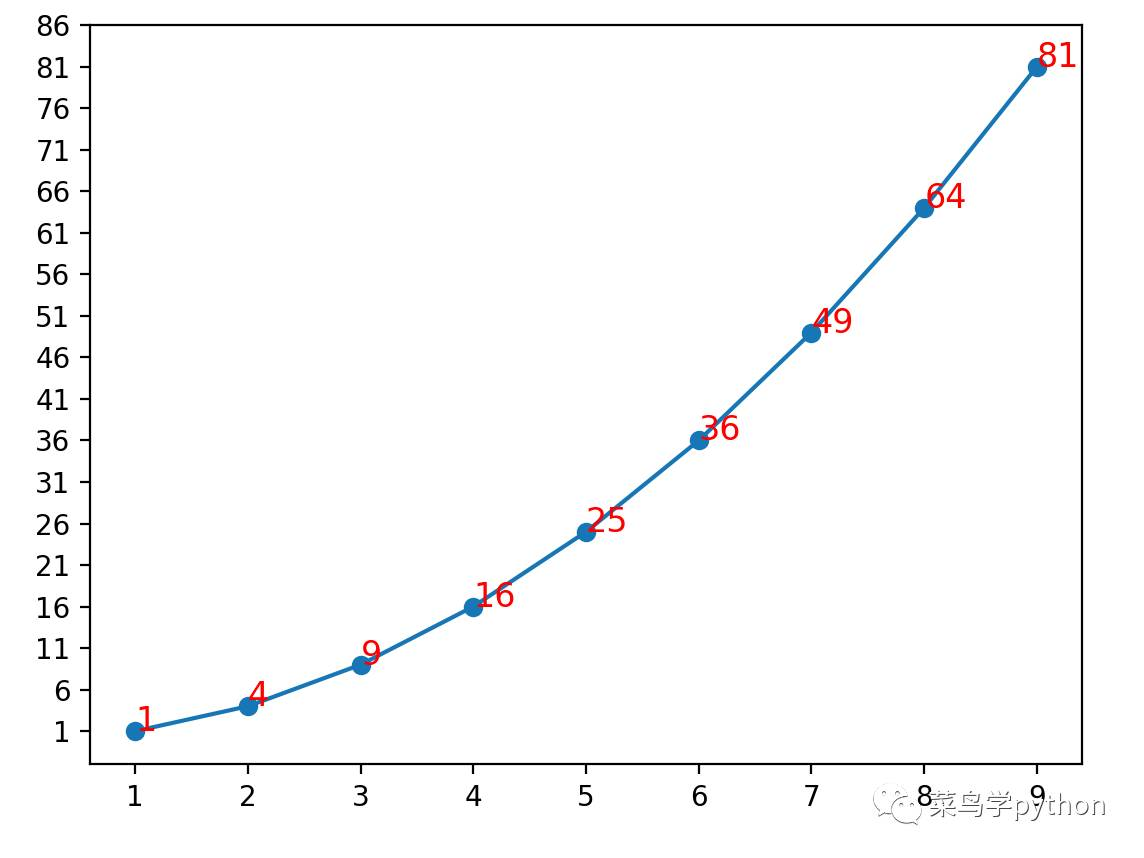是不是很简单啊，有没有更花色的玩法呢：不急我把常见的色彩和样式都总结好了，接着看3.花色折线图下面是我总结的常见的折线图的色彩和样式，然后我们用一个字典来综合秀一把,花色大全折线图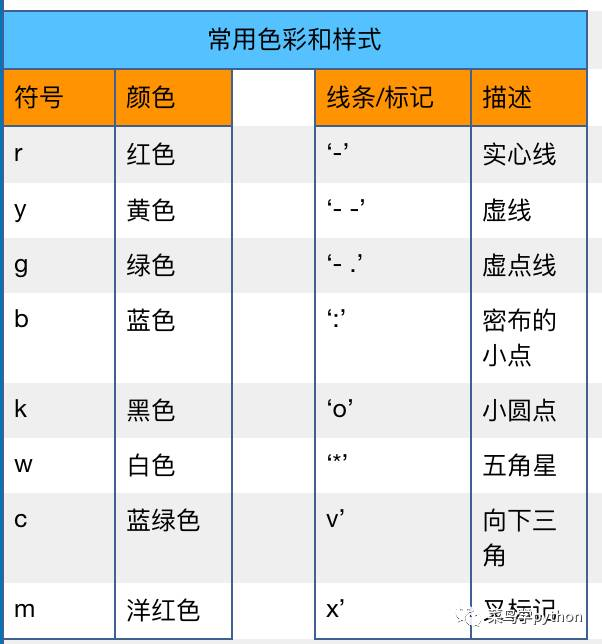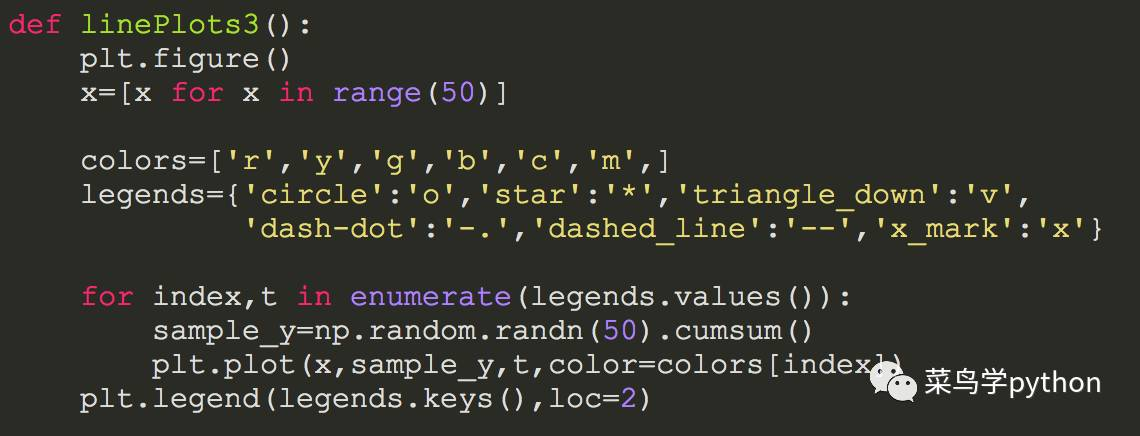x坐标:我们用[0，50]序列为x轴的值y坐标:这次我们用numpy里面的随机函数，随机产生50个的正态分布的样本值然后构造一个字典把常见的类型和描述全部录入,plt.plot()画出曲线最后plt.legend()在左上角显示图例说明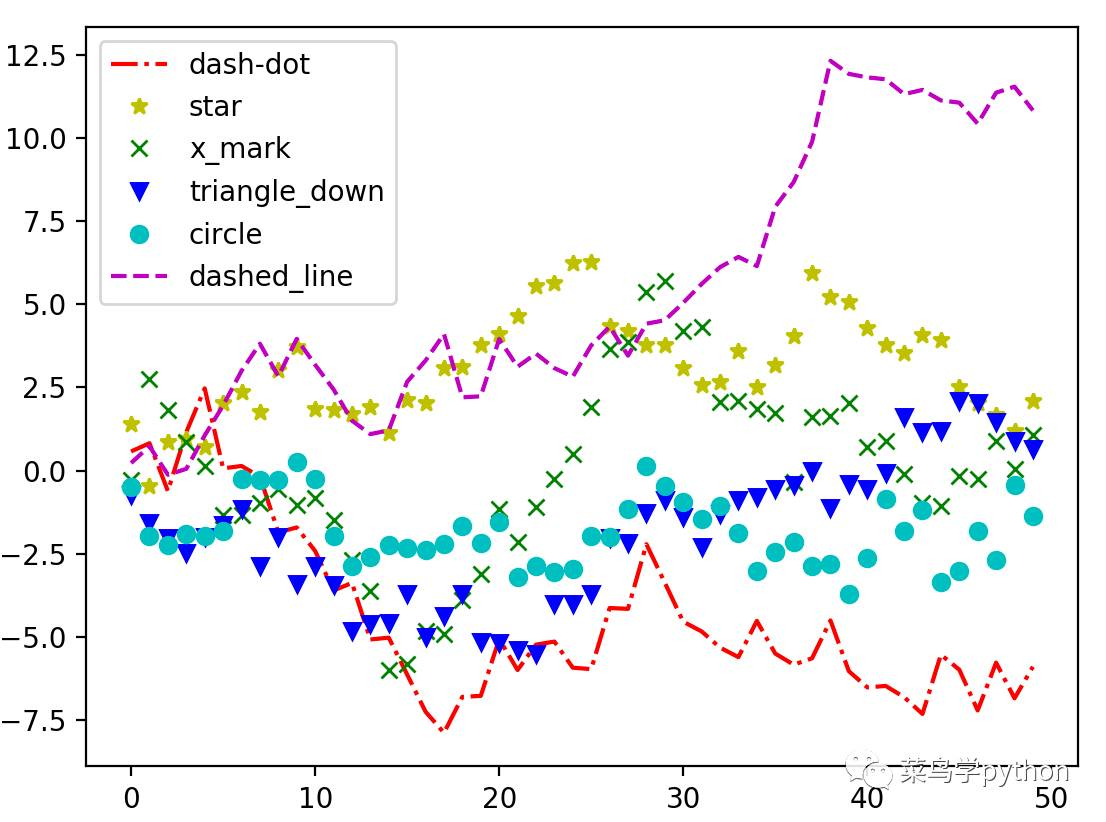这么复杂的图形和曲线，如果我们有几条曲线在图上，想知道曲线之间的面积如何做呢，我们接着看4.带阴影区的折线图阴影区其实就是获取axes对象，然后巧妙的用fill_between()这个函数进行填充，比如我们有3条曲线：x的平方，x的立方和x的4次方，用填充区可以看的更直观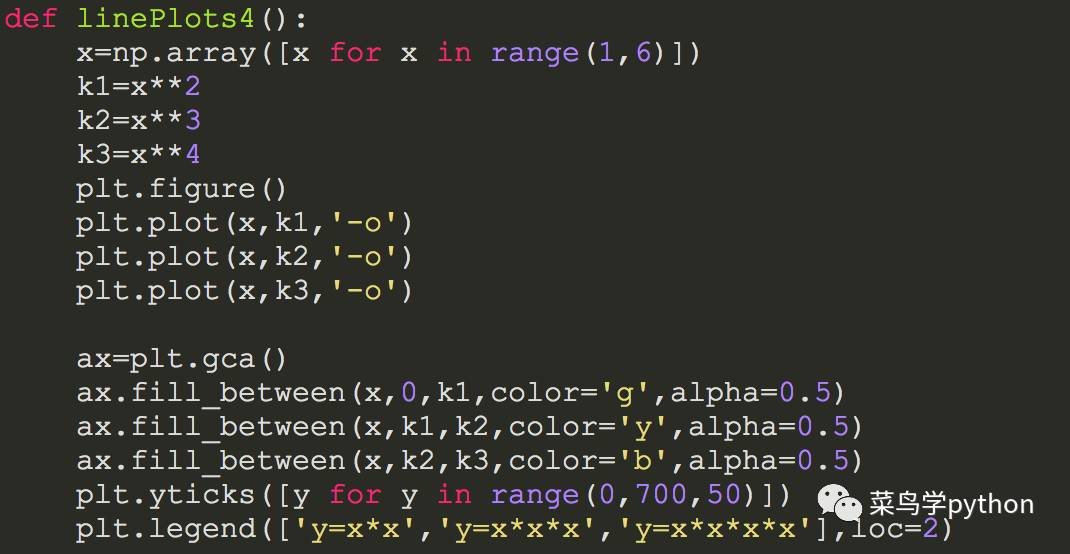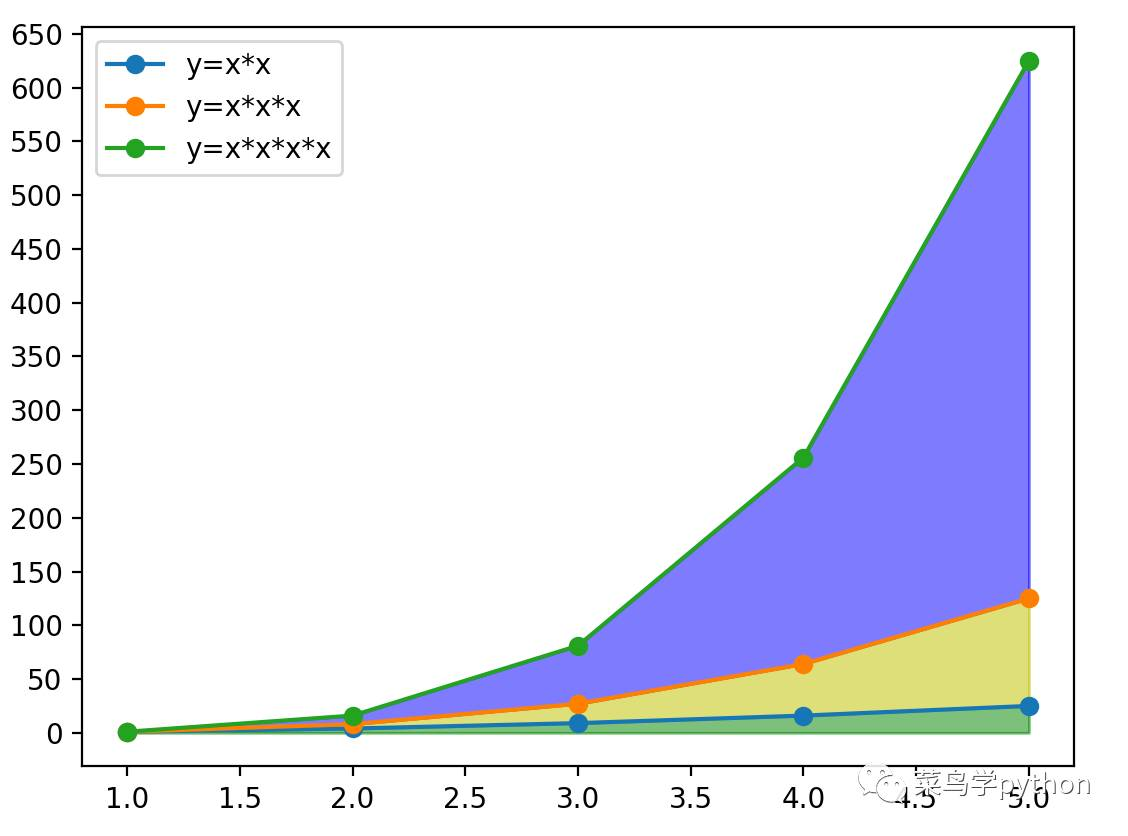综合练习，画个天气预报上面讲了这么多，我们用一个综合的小例子来把上面学的东西融合一下。画一个天气预报的图，我们希望能看到温度的变化,风力的变化，横坐标我们用日期，纵坐标有数字表示温度和风力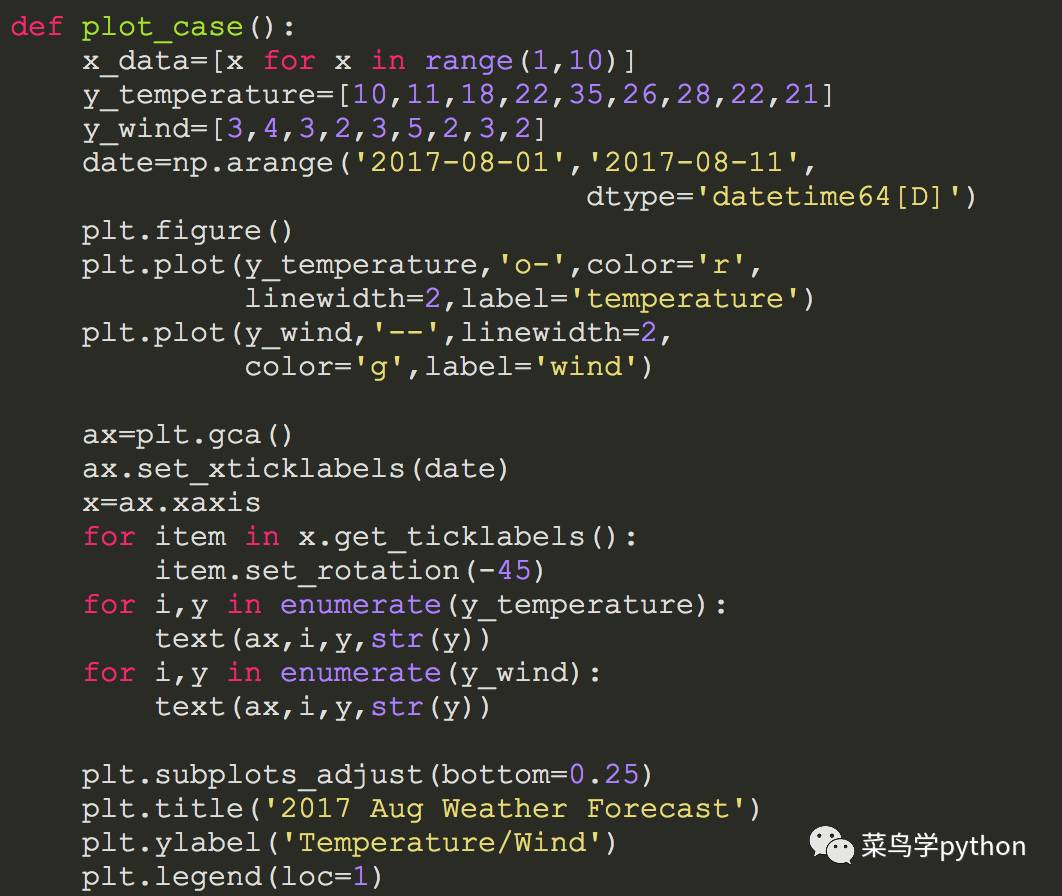先初始化x轴和y轴的参数(y轴有两个参数曲线)把风力和温度的曲线画出来把x轴的坐标用日期表示获得xaxis的对象，循环把风力和温度的数值标出来最后调整一下画布的布局和加上x,y轴的label和图例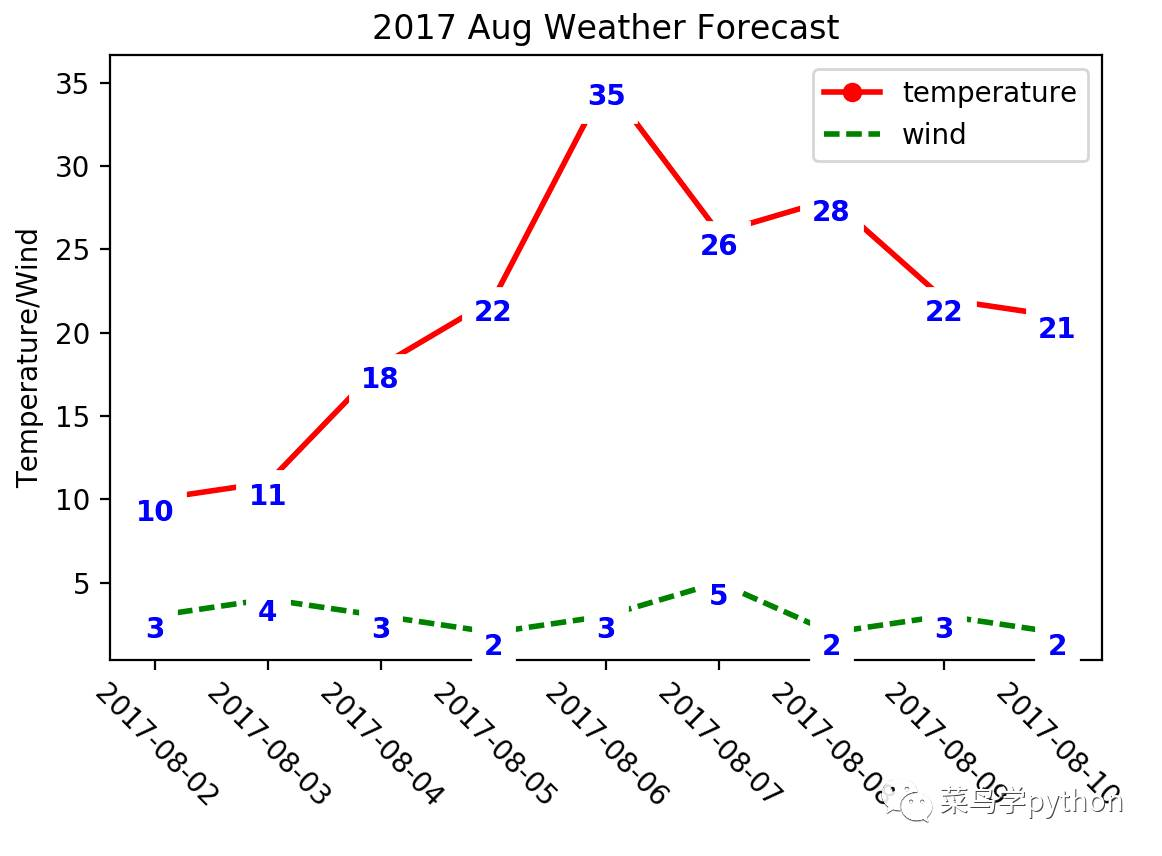结论:好，数据可视化神器matplotlib之折线图篇，就讲到这里，是不是觉得还蛮简单的，不要光说不练，小伙伴们动起手来，若有什么问题，欢迎大家留言，一起讨论，后面还有很多好玩的图，敬请期待另外:全网Python书爬取的源码，有一点问题，采用cookie和延迟的办法，并不能完全绕过网站的反爬虫，会被禁IP.需要用代理池才能彻底解决.最近我在研究数据可视化, 实在没有时间优化，需要源码的同学可以留言，我把CSV文件和数据库查询源码先给大家欢迎大家关注 菜鸟学Python",更多好玩有趣的Python原创教程,趣味算法,经验技巧,行业动态，尽在菜鸟学Python,一起来学python吧量化交易人气文章来源 | 菜鸟学Python作者 | xinxin本文章为菜鸟学Python独家原创稿件，未经授权不得转载责任编辑：
展开全文• 测试开发技术——python折线图生成前言关于分享立竿见影的使用体验python图表生成技术对比循序渐进的查看过程逐步深入到设计过程 前言 测试开发技术中，数据处理技术在我们大量的测试数据，测试结果自动化生成，自动...机器学习 数据分析
• 说起折线图，很多人都觉得非常简单，不就是一些点连成的线吗？用 Excel 几秒钟就能画出一张折线图。真的就这么简单吗？想一想：在普通的折线图中，如何自动地添加一条代表平均值的横线？如何添加一条带箭头的趋势线...
• 利用plt中plot绘制折线图的代码 import pandas as pd import os os.chdir("C:\\Users\Administrator.LAPTOP-1HM8PV0L\Desktop\论文\论文数据\描述性统计分析") table=pd.read_table("全国层面泰尔指数、indl1、indl2...
• 原标题：最适合小白学的花色玩Python折线图|画个天气预报欢迎关注天善智能 hellobi.com，我们是专注于商业智能BI，大数据，数据分析领域的垂直社区，学习、问答、求职，一站式搞定！对商业智能BI、大数据分析挖掘、...
• import matplotlib.pyplot as plt import numpy as np if __name__ == '__main__': pm_15db_3p = [302, 345, 365, 405, 425] pm_20db_3p = [312, 356, 375, 417, 439] pm_15db_rd = [200, 239, 256, 300, 320]...
• 第一篇博客，以我的真实数据总结一下2020年！ 前言 今年是很值得记录的一年，上半年在家大半年，由于自律运动锻炼体重下降到了史上最低值，自返校后，我就尽量每天晚上记录体重，年末，我就用体重值和自己的学的数据...数据分析 matplotlib...

# python折线图python 订阅